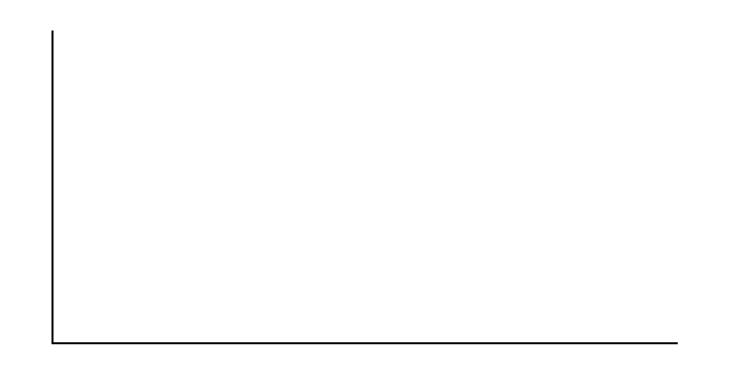Linear Regression and Correlation

# Regression (Distance from School)

Regression (Distance from School)

Class Time:

Names:

Student Learning Outcomes
• The student will calculate and construct the line of best fit between two variables.
• The student will evaluate the relationship between two variables to determine if that relationship is significant.

Collect the DataUse eight members of your class for the sample. Collect bivariate data (distance an individual lives from school, the cost of supplies for the current term).

1. Complete the table.
Distance from school Cost of supplies this term
2. Which variable should be the dependent variable and which should be the independent variable? Why?
3. Graph “distance” vs. “cost.” Plot the points on the graph. Label both axes with words. Scale both axes.Analyze the DataEnter your data into your calculator or computer. Write the linear equation, rounding to four decimal places.

1. Calculate the following:
1. a = ______
2. b = ______
3. correlation = ______
4. n = ______
5. equation: ŷ = ______
6. Is the correlation significant? Why or why not? (Answer in one to three complete sentences.)
2. Supply an answer for the following senarios:
1. For a person who lives eight miles from campus, predict the total cost of supplies this term:
2. For a person who lives eighty miles from campus, predict the total cost of supplies this term:
3. Obtain the graph on your calculator or computer. Sketch the regression line.Discussion Questions
1. Answer each question in complete sentences.
1. Does the line seem to fit the data? Why?
2. What does the correlation imply about the relationship between the distance and the cost?
2. Are there any outliers? If so, which point is an outlier?
3. Should the outlier, if it exists, be removed? Why or why not?